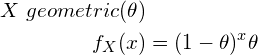# Negative Binomial Distribution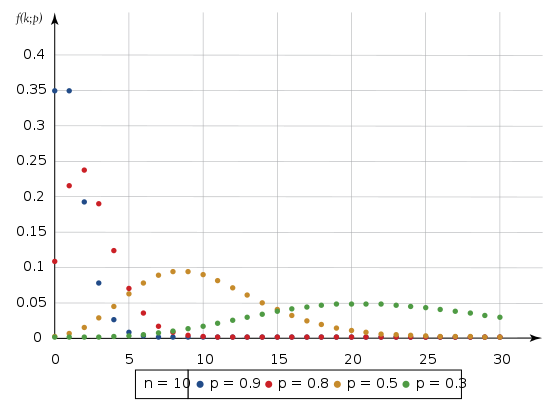## Introduction

Negative Binomial Distribution (also known as Pascal Distribution) should satisfy the following conditions;

1. The experiment should consist of a sequence of independent trials.
2. Each trial should have only 2 outcomes. That is Success (S) or Failure (F).
3. Success Probability θ should be constant from trial to trial.
4. The experiment should be continued until the occurrence of r total successes. Here r is a specified positive integer.

In the Binomial Distribution, we were interested in the number of Successes in n number of trials. But in the Negative Binomial Distribution, we are interested in the number of Failures in n number of trials. This is why the prefix “Negative” is there.

## Detailed Example – 1

Consider an experiment where we roll a die until the face 6 turns upwards two times.

Here;

1. Our trials are independent. That means turning 6 face upwards on one trial does not affect whether or 6 face turns upwards on the next trials.
2. Each trial only has 2 outcomes.
3. Success probability is constant. 1/6 for every trial.
4. The experiment is continued until the 6 face turns upwards 2 times.

## Probability Distribution

#### X = Number of failures that precede the rth success.

(1)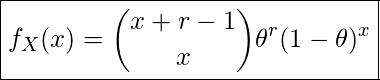Terminology;

• x – value of the Random Variable X
• r – rth success. (no. of failures = (r-1))
• θ – Success probability

## Detailed Example – 2

A researcher is interested in examining the relationship between students’ mental health and their exam marks. For this, he wishes to conduct interviews with 5 students.

θ = Probability of a randomly selected student agrees to sit for the interview

θ = 0.2

What is the probability that 15 students should be asked before 5 students are found to agree to sit for the interview?

(2)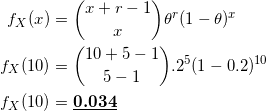## Expected Value and Variance

Expected number of trials until first success is;

(3)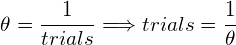Therefore, expected number of failures until first success is;

(4)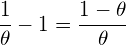Hence, we expect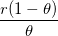failures before the rth success.

Therefore,

(5)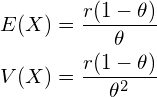## Geometric Distribution

This is a special case of Negative Binomial Distribution where r=1

That means, we are interested in finding number of trials that is required for a single success.

Example :
Tossing a coin until it lands on heads.

#### Formula

(6)